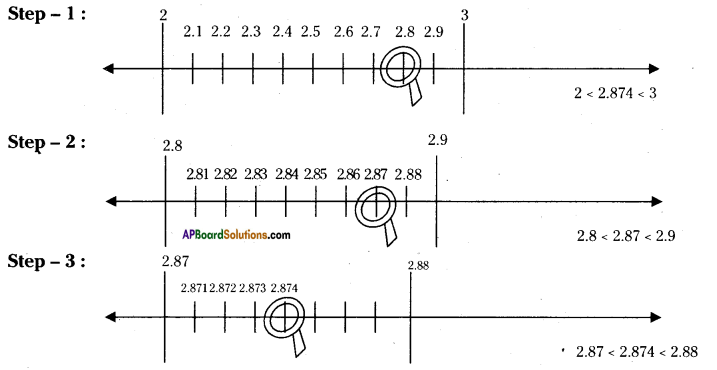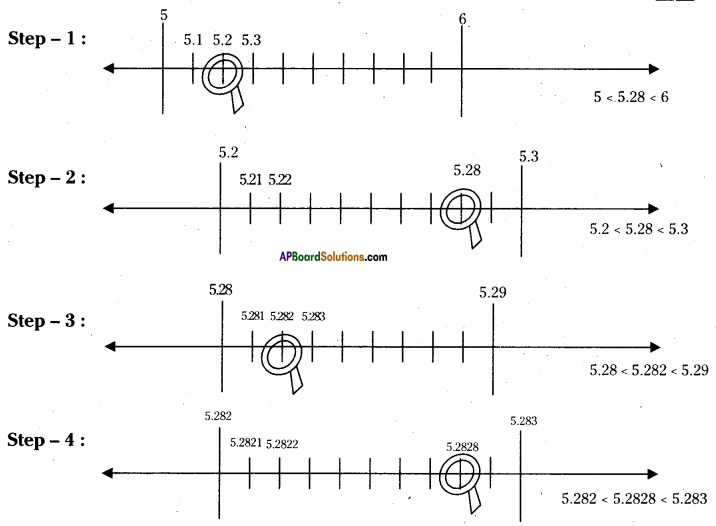AP State Syllabus AP Board 9th Class Maths Solutions Chapter 1 Real Numbers Ex 1.3 Textbook Questions and Answers.

## AP State Syllabus 9th Class Maths Solutions 1st Lesson Real Numbers Exercise 1.3

Question 1.
Visualise $$2.8 \overline{74}$$ on the number line, using successive magnification.
Solution:Question 2.
Visualise $$5 . \overline{28}$$ on the number line, upto 3 decimal places.
Solution: